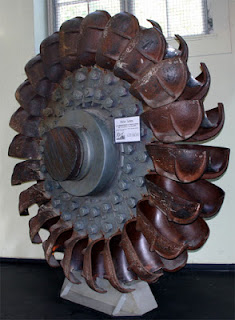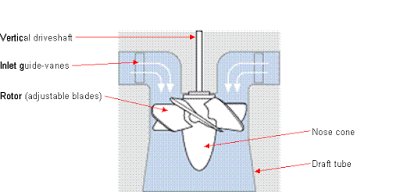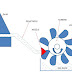Today we will learn about what is turbine and types of turbines used in mechanical industries. We have heard many time the word “turbine”, but most of us don’t know more about It. Turbine is a device which converts the kinetic energy of the fluid into mechanical energy. The fluid may be either water or gas or steam according to the availability. It is mainly used in power plant industries to generate electric power. It can be categorized into three types. The first one is known as the hydraulic turbine, the second one is the gas turbine and the last one is the steam turbine.
Hydraulic turbine is hydraulic machines which convert hydraulic energy of water into mechanical energy. It installed at the dam or near any large water reservoir.
Gas turbine uses compressed air or other gases to rotate the turbine and convert the kinetic energy of gases into mechanical energy.
The last one is a steam turbine in which first water is converted into high-pressure superheated steam, which is further used to drive the turbine.

## What is turbine?

A hydraulic machine which converts hydraulic energy into
mechanical energy is known as the turbine. Turbine is a device which converts the kinetic energy of water, air, gas or steam into mechanical energy which is further used to rotate the generator shaft. Simply turbine is a rotor which has vanes at the peripheral and connected at the end of an electromagnet or well-known generator shaft which causes to rotate it and generate electric power.

We know that the turbine is mostly used in hydroelectric power
plants. As shown in diagram a dam is constructed across a river to store water. There
is an elevation difference between turbine and dam. Turbine and dam are connected
by a large diameter pipe called penstock which carries pressurize water from the storage reservoir to the turbine. The turbine is connected to an electromagnet. When water strikes the turbine blades, it makes rotate the turbine hence electric generator which produces electric power. Turbine has different types of vanes fitted on rotter. The water is carried to tailrace after worked on the
turbine.

## Types of Turbines:

Turbines can be classified in various ways like according to the direction of the fluid, head at the inlet of the turbine, types of fluid etc. These
are

### According to the type of energy at the inlet:

1. Impulse turbine:

If a turbine uses only the impulse energy of the fluid, it is known as the impulse turbine. The impulse energy means it uses the only kinetic energy of the fluid. In this type of turbine, first whole pressure energy or head converted into kinetic energy and this kinetic energy used to rotate the turbine. The water uses only its kinetic energy and the pressure of water remains constant at the inlet and exit of it.
Example: Pelton WheelPelton Wheel Turbine

2. Reaction turbine:

If the water pressure at the inlet and exit of
the turbine is different or the water use sits kinetic energy as well as pressure
energy to rotate the turbine, is known as reaction turbine. When the water under
pressure flows through the turbine, some of its pressure energy converts into
kinetic energy which rotate the turbine.
Example: Francis TurbineKaplan Turbine (Reaction Turbine)

### According to the direction of flow through runner:

1. Tangential flow turbine:
If the water flows along the tangent of the runner, the
turbine is known as tangential flow turbine. Pelton wheel turbine is the
example of tangential flow turbine.
If the water flows in the radial direction through the runner,
the turbine is called the radial flow turbine. If the water flows from outward to
inward, radially, the turbine is known as the inward radial flow turbine and if oppose
the turbine is known as an outward radial flow turbine.
3. Axial flow turbine:
If the water flows along the direction parallel to the axis
of the runner, the turbine is known as axial flow turbine. Kaplan Turbine is the
example of an axial flow turbine.
4. Mixed flow turbine:
If the water flows through the runner in the radial direction
but leaves in the direction parallel to the axis of rotation of the runner, the
turbine is called a mixed flow turbine. Francis is the example of mixed flow turbine.

### According to the head at the inlet of the turbine:

What is Turbine? What Are Main Types of Turbines?Reviewed by Admin on July 26, 2018 Rating: 5